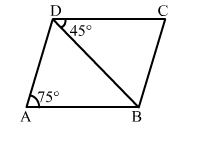# In the given figure, ABCD is a parallelogram in which ∠BDC = 45°

Question:

In the given figure, ABCD is a parallelogram in which BDC = 45° and ∠BAD = 75°. Then, ∠CBD = ?
(a) 45°
(b) 55°
(c) 60°
(d) 75°Solution:

(c)​ 60°

Explanation:
∠BAD = ∠BCD = 75o       [Opposite angles are equal]
In ∆ BCD, ∠ C = 75o
∴ ​∠CBD = 180o  (75o + 45o) = 60o